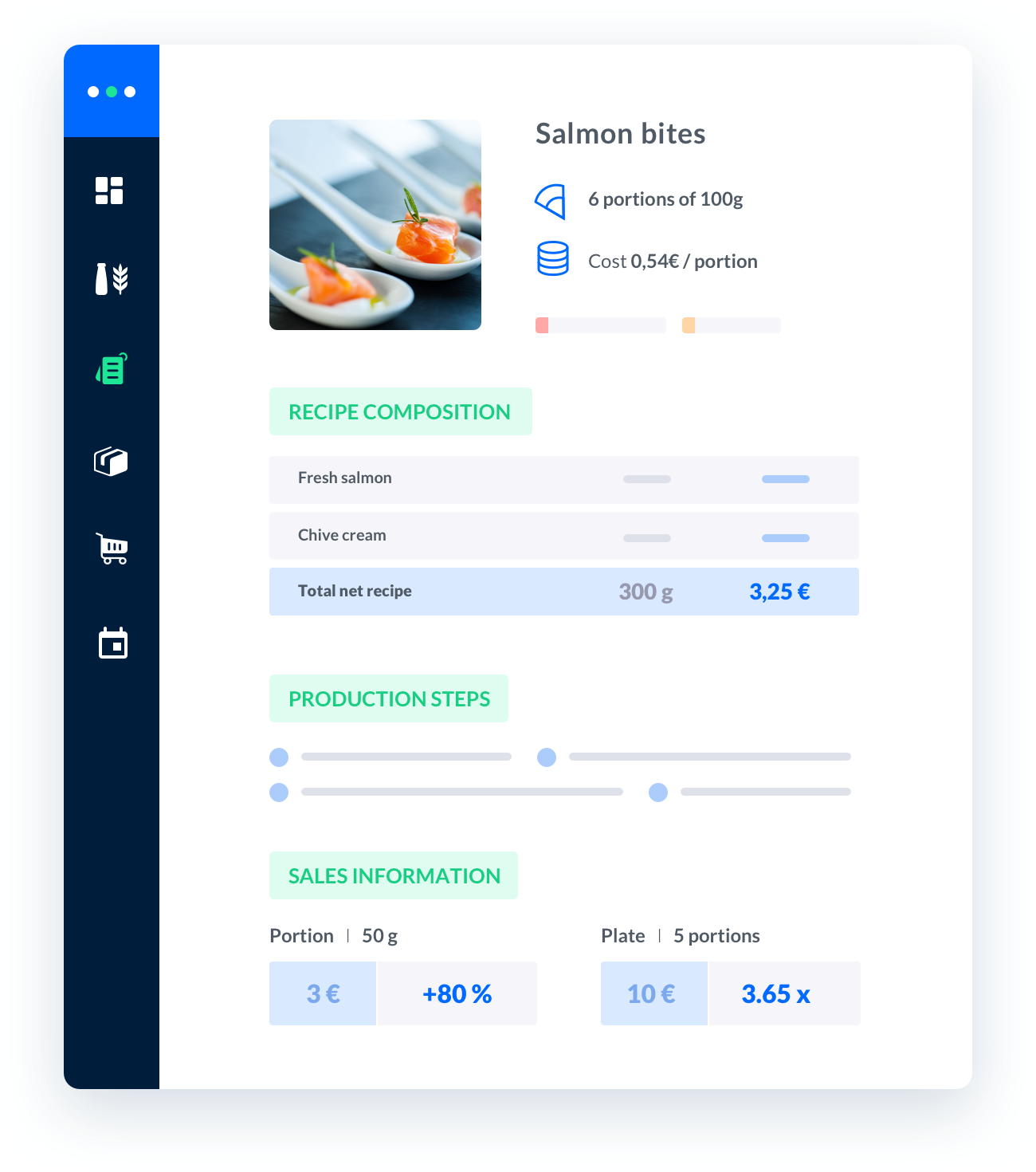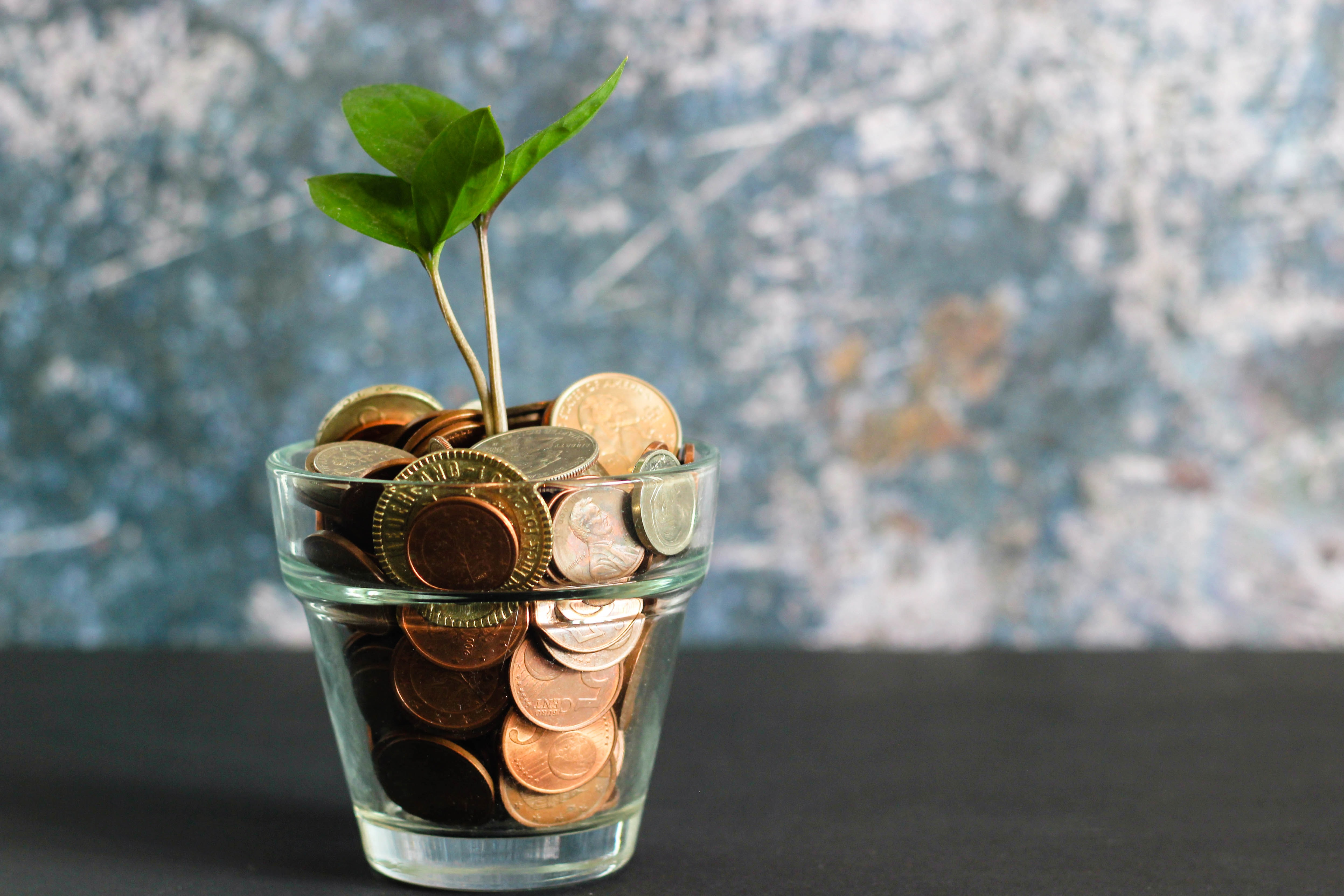# percentage cost formula

Calculating Percentage Cost.Calculating the percentage cost of a product or service is an important part of running a successful business.

Published on 01/01/2023Sébastien Vassaux##### Melba: the food cost app to optimize the profitability of your restaurant

Discover how to optimize the profitability of your restaurant with melba## Calculating Percentage Cost

Calculating the percentage cost of a product or service is an important part of running a successful business. Knowing the percentage cost of a product or service can help you determine the profitability of your business.

## Formula for Calculating Percentage Cost

The formula for calculating percentage cost is as follows:

• Percentage Cost = (Cost of Goods Sold / Revenue) x 100

The cost of goods sold is the total cost of the product or service, including materials, labor, and overhead. Revenue is the total amount of money received from the sale of the product or service.

## Example of Calculating Percentage Cost

Let's say you sell a product for \$100. The cost of goods sold for that product is \$50. The percentage cost of the product would be calculated as follows:

• Percentage Cost = (\$50 / \$100) x 100 = 50%

This means that the cost of the product is 50% of the total revenue.

## Conclusion

Calculating the percentage cost of a product or service is an important part of running a successful business. Knowing the percentage cost of a product or service can help you determine the profitability of your business.

## Take back control over your kitchen

Subscribe to our disrupting service to boost your productivity and profitability